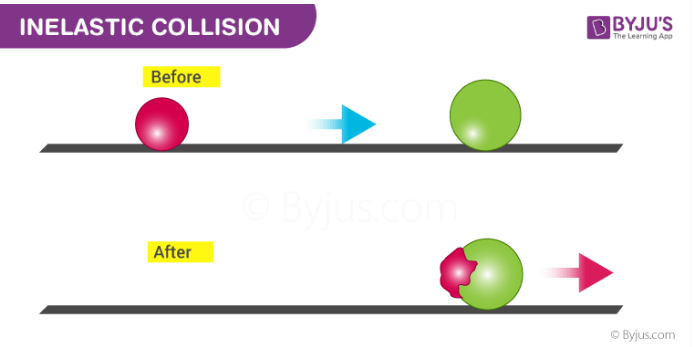# Perfectly Inelastic Collision MCQs for NEET

In inelastic collision momentum is conserved but kinetic energy is not conserved. A perfectly inelastic collision is such a type of collision that takes place between two objects where the two objects stick to each other after the collision. Example, when a wet mudball is thrown against a wall mudball stick to the wall.Q1: The coefficient of restitution e for a perfectly inelastic collision is

1. 1
2. 0
3. Infinity
4. -1

Q2: When two bodies stick together after the collision, the collision is said to be

1. Partially elastic collision
2. Totally elastic collision
3. Totally inelastic collision
4. None of the above

Q3: A completely inelastic collision is one in which the two colliding particles

1. Are separated after collision
2. Remain together after the collision
3. Split into small fragments flying in all directions
4. None of the above

Answer: (b) Remain together after the collision

Q4: A bullet hits and gets embedded in a solid block resting on a horizontal frictionless table. What is conserved?

1. Momentum and kinetic energy
2. Kinetic energy alone
3. Momentum alone
4. Neither momentum nor kinetic energy

Q5: A body of mass m moving with a constant velocity v hits another body of the same mass moving with the same velocity v but in the opposite direction and sticks to it. The velocity of the compound body after the collision is

1. 2v
2. v/2
3. V
4. Zero

Q6: A 50 g bullet moving with velocity 10 m/s strikes a block of mass 950 g at rest and gets embedded in it. The loss in kinetic energy will be

1. 100%
2. 95%
3. 5%
4. 50%

Q7: A ball is dropped from a height 10m. The ball is embedded in sand 1m and stops, then

1. Only momentum remains conserved
2. Only kinetic energy remains conserved
3. Both momentum and K.E are conserved
4. Neither K.E nor momentum is conserved

Answer: (a) Only momentum remains conserved

Q8: Which of the following is not perfectly inelastic collision?

1. Striking of two glass balls
2. A bullet striking a bag of sand
3. An electron captured by a proton
4. A man jumping onto a moving cart

Answer: (c) An electron captured by a proton

Q9: A particle of mass m moving with velocity v strikes a stationary particle of mass 2m and stricks to it. The speed of the system will be

1. v/2
2. 2v
3. v/3
4. 3v

Q10: If a skater of weight 3 Kg has initial speed 32 m/s and the second one of weight 4 kg has 5m/s. After the collision, they have speed (couple) 5 m/s. Then the loss in K.E is

1. 48 J
2. 96 J
3. Zero
4. None of these